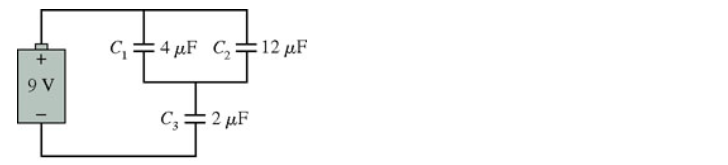# Problem: What are the charge on and the potential difference across each capacitor in the figure ?Part A. Q1Part B. V1Part C. Q2Part D. V2Part E. Q3Part F. V3

###### FREE Expert Solution

For 2 capacitors in series, the equivalent capacitance is:

$\overline{){{\mathbf{C}}}_{\mathbf{e}\mathbf{q}}{\mathbf{=}}\frac{{\mathbf{C}}_{\mathbf{1}}{\mathbf{C}}_{\mathbf{2}}}{{\mathbf{C}}_{\mathbf{1}}\mathbf{+}{\mathbf{C}}_{\mathbf{2}}}}$

For capacitors in parallel, the equivalent capacitance is:

$\overline{){{\mathbf{C}}}_{\mathbf{e}\mathbf{q}}{\mathbf{=}}{{\mathbf{C}}}_{{\mathbf{1}}}{\mathbf{+}}{{\mathbf{C}}}_{{\mathbf{2}}}{\mathbf{+}}{\mathbf{.}}{\mathbf{.}}{\mathbf{.}}{\mathbf{+}}{{\mathbf{C}}}_{{\mathbf{n}}}}$

The charge stored by a capacitor:

$\overline{){\mathbf{Q}}{\mathbf{=}}{\mathbf{C}}{\mathbf{V}}}$

From the figure, C1 and C2 are in parallel.

Therefore, C12 = C1 + C2 = 4 + 12 = 16 μF

C12 and C3 are in series.

85% (484 ratings)###### Problem Details

What are the charge on and the potential difference across each capacitor in the figure ?

Part A. Q1
Part B. V1
Part C. Q2
Part D. V2
Part E. Q3
Part F. V3